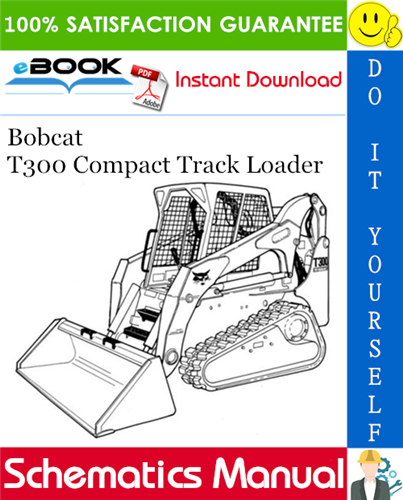Complete WIRING/HYDRAULIC/HYDROSTATIC SCHEMATIC for the Bobcat T300 Compact Track Loader.

This manual contains high quality images, Schematics and instructions to help you to operate and repair your Machine.

Covers the following serial numbers:
=========
T300 (S/N A3GU20001 AND ABOVE)
T300 (S/N A3GV20001 AND ABOVE)
T300 (S/N A5GU20001 AND ABOVE)
T300 (S/N A5GV20001 AND ABOVE)
T300 (S/N A5GU11001 – A5GU19999)
T300 (S/N A5GV11001 – A5GV19999)
T300 (S/N 532011001 AND ABOVE)
T300 (S/N 532111001 AND ABOVE)
T300 (S/N 532015978 AND ABOVE)
T300 (S/N 532111535 AND ABOVE)
T300 (S/N 532111513 AND ABOVE)
T300 (S/N 532013988 AND ABOVE)
T300 (S/N 532111170 AND ABOVE)
T300 (S/N 532011001 – 532013987)
T300 (S/N 532111001 – 532111169)
T300 (S/N 532011001 – 532015977)
T300 (S/N 532111001 – 532111534)
T300 (S/N 532011392 – 532013987)
T300 (S/N 532111013 – 532111169)
T300 (S/N 532011001 – 532011391)
T300 (S/N 532111001 – 532111012)
T300 (S/N 532011001 – 532013987)
T300 (S/N 532111001 – 532111169)
T300 (S/N 525414000 & Above)
T300 (S/N 525512000 & Above)
T300 (S/N 525411001 AND ABOVE)
T300 (S/N 525511001 AND ABOVE)
T300 (S/N 525411001 – 525413999)
T300 (S/N 525511001 – 525511999)
T300 (S/N 521011601 & Above)
T300 (S/N 522011103 & Above)
T300 (S/N 521911001 – 521011600)
T300 (S/N 522011001 – 522011102)
T300 (S/N 521911001 AND ABOVE)
T300 (S/N 522011001 AND ABOVE)

=========

Model Specification: Bobcat T300 Compact Track Loader
Language: English
File Format: PDF